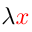Higher-Order Logic Explorer < Previous   Next > Nearby theorems Mirrors  >  Home  >  HOLE Home  >  Th. List  >  ax-ceq GIF version

Axiom ax-ceq 46
 Description: Equality theorem for combination.
Hypotheses
Ref Expression
ax-ceq.1 F:(αβ)
ax-ceq.2 T:(αβ)
ax-ceq.3 A:α
ax-ceq.4 B:α
Assertion
Ref Expression
ax-ceq ((( = F)T), (( = A)B))⊧(( = (FA))(TB))

Detailed syntax breakdown of Axiom ax-ceq
StepHypRef Expression
1 ke 7 . . . . 5 term =
2 tf . . . . 5 term F
31, 2kc 5 . . . 4 term ( = F)
4 tt . . . 4 term T
53, 4kc 5 . . 3 term (( = F)T)
6 ta . . . . 5 term A
71, 6kc 5 . . . 4 term ( = A)
8 tb . . . 4 term B
97, 8kc 5 . . 3 term (( = A)B)
105, 9kct 10 . 2 term ((( = F)T), (( = A)B))
112, 6kc 5 . . . 4 term (FA)
121, 11kc 5 . . 3 term ( = (FA))
134, 8kc 5 . . 3 term (TB)
1412, 13kc 5 . 2 term (( = (FA))(TB))
1510, 14wffMMJ2 11 1 wff ((( = F)T), (( = A)B))⊧(( = (FA))(TB))
 Colors of variables: type var term This axiom is referenced by:  eqcomx  47  ceq12  78
 Copyright terms: Public domain W3C validator